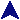Example of Jensen's DeviceCSc 404 Documentation And Examples```begin
comment This is a simple example of Jensen's device;

comment This takes the sum of expr from start to stop, inclusive.
The variable index is incremented through the range.;
real procedure sumof(start, stop, index, expr);
value start, stop;
integer start, stop, index;
real expr;
begin
real sum;

comment initialize sum to zero and add the terms.;
sum := 0.0;
for index := start step 1 until stop do begin
sum := sum + expr
end;

sumof := sum
end;

begin
comment Sum of integers, 1 to 10.;
integer i;
outreal(1, sumof(1, 10, i, i));
outstring(1, "\n");
end;

begin
comment sum of squares, -5 to 5.;
integer j;
outreal(1, sumof(-5, 5, j, j*j));
outstring(1, "\n");
end;
end
```